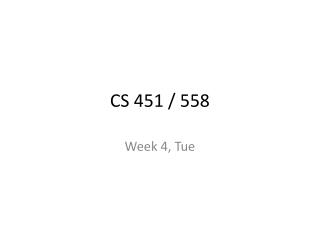DownloadDownload PresentationCS 451 / 558

# CS 451 / 558

Télécharger la présentation## CS 451 / 558

- - - - - - - - - - - - - - - - - - - - - - - - - - - E N D - - - - - - - - - - - - - - - - - - - - - - - - - - -
##### Presentation Transcript

1. CS 451 / 558 Week 4, Tue

2. Scoring an alignment Let: xk:= the kthletter of x yk:= the kthletter of y Input: string x, length m string y, length n (both from alphabet S [ACGT]) scoring matrix s, s.t.s(a,b) := the score of aligning a to b gap penalty g S = 0 # the score for (i=0; i<m; i++) if (Si or Ti is a gap character) S -= g else S += s(xi ,yi)

3. Finding an optimal alignment Dynamic Programming • Looks like a merger of the 2D dotplot array and the alignment scoring

4. Finding an optimal alignment Dynamic Programming • Looks like a merger of the 2D dotplot array and the alignment scoring • But it’s actually more than that

5. Finding an optimal alignment Dynamic Programming • a recursive definition of the optimal score

6. a recursive definition of the optimal score the optimal solution depends on • optimal solutions to subproblems of the same form • local calculations based on those solutions • e.g. score for alignment of x and y • Can only end one of three ways: • xm aligned to yn • xm aligned to nothing (ynalready used) • yn aligned to nothing (xmalready used) S = S(m-1,n-1) + s(xm,yn) S = S(m-1,n) + g S = S(m,n-1) + g

7. a recursive definition of the optimal score the optimal solution depends on • optimal solutions to subproblems of the same form • local calculations based on those solutions • e.g. score for alignment of x and y • Can only end one of three ways: • xm aligned to yn • xm aligned to nothing (ynalready used) • yn aligned to nothing (xmalready used) • generally S = S(m-1,n-1) + s(xm,yn) S = S(m-1,n) + g S = S(m,n-1) + g

8. Finding an optimal alignment Dynamic Programming • a recursive definition of the optimal score

9. Finding an optimal alignment Dynamic Programming • a recursive definition of the optimal score

10. Finding an optimal alignment Dynamic Programming • a recursive definition of the optimal score • a dynamic programming matrix for remembering optimal scores of subproblems

11. Finding an optimal alignment Dynamic Programming • a recursive definition of the optimal score • a dynamic programming matrix for remembering optimal scores of subproblems • a bottom-up approach of filling the matrix by solving the smallest subproblems first

12. a bottom-up approach of filling the matrix by solving the smallest subproblems first move me

13. Scoring an optimal alignment Input: strings x & y, lengths m & n scoring matrix s, s.t.s(a,b) := the score of aligning a to b gap penalty g S0,0 = 0 # the score for (i=0; i<m; i++) initialize Si,0 for (j=0; j<n; j++) initialize S0,j for (i=0; i<m; i++) for (j=0; j<n; j++) Si-1,j-1 +s(xi ,yi), Si,j = max Si,j-1 – g , Si-1,j– g

14. Finding an optimal alignment Dynamic Programming • a recursive definition of the optimal score • a dynamic programming matrix for remembering optimal scores of subproblems • a bottom-up approach of filling the matrix by solving the smallest subproblems first • a traceback of the matrix to recover the structure of the optimal solution that gave the optimal score

15. Global alignment vs local alignment

16. 0 0 0 0 0 0 0 0 0 0 0 0 0 5 0 0 0 0 0 0 0 5 5 0 3 0 0 0 4 2 0 0 3 0 8 10 4 0 8 6 0 2 0 0 2 4 0 2 0 5 12 0 6 0 0 0 2 0 0 6 0 18 0

17. Global alignment vs local alignment

18. local alignment Input: strings x & y, lengths m & n scoring matrix s, s.t.s(a,b) := the score of aligning a to b gap penalty g S0,0 = 0 # the score for (i=0; i<m; i++) initialize Si,0 for (j=0; j<n; j++) initialize S0,j for (i=0; i<m; i++) for (j=0; j<n; j++) Si-1,j-1 +s(xi ,yi), Si,j = max Si,j-1 – g , Si-1,j– g 0

19. Score matrix P(xi , yj| model of homology)

20. Score matrix P(xi , yj | model of homology) P(xi , yj | model of nonhomology)

21. Score matrix f (xi , yj) * f (xi) f (yj) ** * From alignments of trusted homologs ** Observed frequencies in large database of repr. seqs

22. Score matrix f (xi , yj) * s(xi , yj)= log f (xi) f (yj) ** * From alignments of trusted homologs ** Observed frequencies in large database of repr. seqs

23. Score matrix BLOSUM PAM VTML … f (xi , yj) * s(xi , yj)= log f (xi) f (yj) **

24. Score matrix f (xi , yj) * s(xi , yj)= log f (xi) f (yj) **

25. Gap penalties Usually ad hoc What works well with the chosen score matrix Linear / affine gap penalties Affine = geometric length distribution